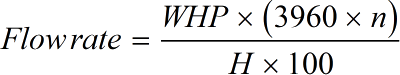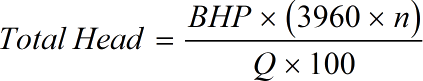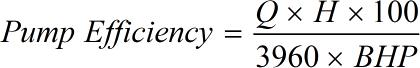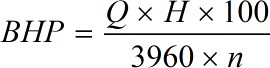Request a Tool

Brake Horsepower Calculator

The advanced online Brake Horsepower Calculator is useful in calculating the brake horsepower of an engine

Flow Rate
0

Formula• WHP = Water Horsepower
• n = Pump Efficiency

Defination / Uses

Brake horsepower is defined as an engine's actual or useful horsepower as measured by a force applied to a friction brake. On a device known as a dynamometer, the horsepower of an engine is measured. The advanced online Brake Horsepower Calculator may be used to calculate an engine's brake horsepower using a formula.

0

Formula• n = Pump Efficiency
• Q = Flow rate
• BHP = Brake Horsepower

Defination / Uses

Brake horsepower is defined as an engine's actual or useful horsepower as measured by a force applied to a friction brake. On a device known as a dynamometer, the horsepower of an engine is measured. The advanced online Brake Horsepower Calculator may be used to calculate an engine's brake horsepower using a formula.

Pump Efficiency
0

Formula• Q = Flow rate
• BHP = Brake Horsepower

Defination / Uses

Brake horsepower is defined as an engine's actual or useful horsepower as measured by a force applied to a friction brake. On a device known as a dynamometer, the horsepower of an engine is measured. The advanced online Brake Horsepower Calculator may be used to calculate an engine's brake horsepower using a formula.

Brake Horsepower
0

Formula• Q = Flow rate
• n = Pump Efficiency

Defination / Uses

Brake horsepower is defined as an engine's actual or useful horsepower as measured by a force applied to a friction brake. On a device known as a dynamometer, the horsepower of an engine is measured. The advanced online Brake Horsepower Calculator may be used to calculate an engine's brake horsepower using a formula.

How calculte the area of a circle?

There are only two basic steps.

• First select the option from dropdown. What you need to calculate.
• Then add the other values in their corresponding fields.

Thats it! You will get your answer in less than a second. Use upper given formula in case of manual calculation.

The advanced online Brake Horsepower Calculator may be used to calculate an engine's brake horsepower using a formula. The dynamometer linked to the driving shaft is known as the Brake Horsepower. It was first used to compare the power output of steam engines to the power output of draught horses, after it was invented by James Watt. Despite the fact that horsepower is not an officially recognised SI unit, it is nonetheless commonly used in industry. For quick calculation use our weetools. No sign-up, registration OR captcha is required to use this tool.